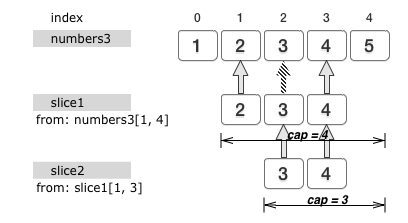## why

• 语法简单，golang 的关键字才 25 个，入门也非常简单
• 天然支持高并发，适用于大型微服务应用
• 跨平台，可编译出支持各大主流平台的应用，且毫无依赖

## how

### 高级数据类型

#### 数组类型

``type MyNumbers int``

``int{1, 2, 3}``

``var numbers = int{1, 2, 3}``

``var numbers = [...]int{1, 2, 3}``

``````numbers // 会得到第一个元素
numbers // 会得到第二个元素
numbers // 会得到第三个元素``````

``numbers = 4``

``var length = len(numbers)``

``var numbers2 int``

``int{0, 0, 0, 0, 0}``

#### 切片类型

``````[]int
// 或
[]string``````

``type MySlice []int``

``[]int{1, 2, 3}``

``````var numbers3 = int{1, 2, 3, 4, 5}
var slice1 = numbers3[1:4]``````

``var slice2 = slice1[1:3]````var capacity2 int = cap(slice2)``

``var slice3 []int``

``numbers3[1:4:4]``

``````var numbers3 = int{1, 2, 3, 4, 5}
var slice1 = numbers3[1:4]``````

slice1 = slice1[:cap(slice1)]

``var slice1 = numbers3[1:4:4]``

``slice1 = append(slice1, 6, 7)``

1. 这种复制遵循最小复制原则，即：被复制的元素的个数总是等于长度较短的那个参数值的长度。
2. 与 append 函数不同， copy 函数会直接对其第一个参数值进行修改。

``````var slice4 = []int{0, 0, 0, 0, 0, 0, 0}
copy(slice4, slice1)``````

#### 字典类型

Go语言的字典（ Map ）类型其实是哈希表（ Hash Table ）的一个实现。字典用于存储键-元素对（更通俗的说法是键-值对）的无序集合。

``map[int]string{1: "a", 2: "b", 3: "c"}``

``mm := map[int]string{1: "a", 2: "b", 3: "c"}``

``b := mm``

``mm = b + "2"``

``````d := mm
e := mm``````

``e, ok := mm``

``delete(mm, 4)``

#### 通道类型

Goroutine（也称为 Go 程序）可以被看做是承载可被并发执行的代码块的载体。它们由 Go 语言的运行时系统调度，并依托操作系统线程（又称内核线程）来并发地执行其中的代码块。

``make(chan int, 5)``

``ch1 := make(chan string, 5)``

``ch1 <- "value1"``

``value := <- ch1``

``value, ok := <- ch1``

``close(ch1)``

``make(chan int, 0)``

``type Receiver <-chan int``

``type Sender chan<- int``

``````var myChannel = make(chan int, 3)
var sender Sender = myChannel

``var myChannel1 chan int = sender``

#### 函数

``func(input1 string ,input2 string) string``

``type MyFunc func(input1 string ,input2 string) string``

``````func myFunc(part1 string, part2 string) (result string) {
result = part1 + part2
return
}``````

``````func myFunc(part1 string, part2 string) string {
return part1 + part2
}``````

``var splice func(string, string) string // 等价于 var splice MyFunc``

``splice = myFunc``

``splice("1", "2")``

``````var splice = func(part1 string, part2 string) string {
return part1 + part2
}``````

``````var result = func(part1 string, part2 string) string {
return part1 + part2
}("1", "2")``````

#### 结构体和方法

Go 语言的结构体类型（ Struct ）比函数类型更加灵活。它可以封装属性和操作。前者即是结构体类型中的字段，而后者则是结构体类型所拥有的方法。

``````type Person struct {
Name   string
Gender string
Age    uint8
}``````

``Person{Name: "Robert", Gender: "Male", Age: 33}``

``Person{"Robert", "Male", 33}``

``````p := struct {
Name   string
Gender string
Age    uint8
}{"Robert", "Male", 33}``````

``````func (person *Person) Grow() {
person.Age++
}``````

``````p := Person{"Robert", "Male" 33}
p.Grow()``````

#### 接口

``````type Animal interface {
Grow()
Move(string) string
}``````

``Move(new string) (old string)``

*Person 类型（注意，不是Person类型）拥有一个 Move 方法。该方法会是Animal接口的 Move 方法的一个实现。再加上我们在之前为它编写的那个 Grow 方法，*Person类型就可以被看做是 Animal 接口的一个实现类型了。

Go 语言的类型转换规则定义了是否能够以及怎样可以把一个类型的值转换另一个类型的值。另一方面，所谓空接口类型即是不包含任何方法声明的接口类型，用 interface{} 表示，常简称为空接口。正因为空接口的定义，Go 语言中的包含预定义的任何数据类型都可以被看做是空接口的实现。我们可以直接使用类型转换表达式把一个*Person类型转换成空接口类型的值，就像这样：

``````p := Person{"Robert", "Male", 33, "Beijing"}
v := interface{}(&p)``````

``h, ok := v.(Animal)``

#### 指针

``````type Person struct {
Name    string
Gender  string
Age     uint8
}

func (person *Person) Grow() {
person.Age++
}

func (person *Person) Move(newAddress string) string {
return old
}``````

``````func (person Person) Grow() {
person.Age++
}``````

``````p := Person{"Robert", "Male", 33, "Beijing"}
p.Grow()
fmt.Printf("%v\n", p)
``````

（完）